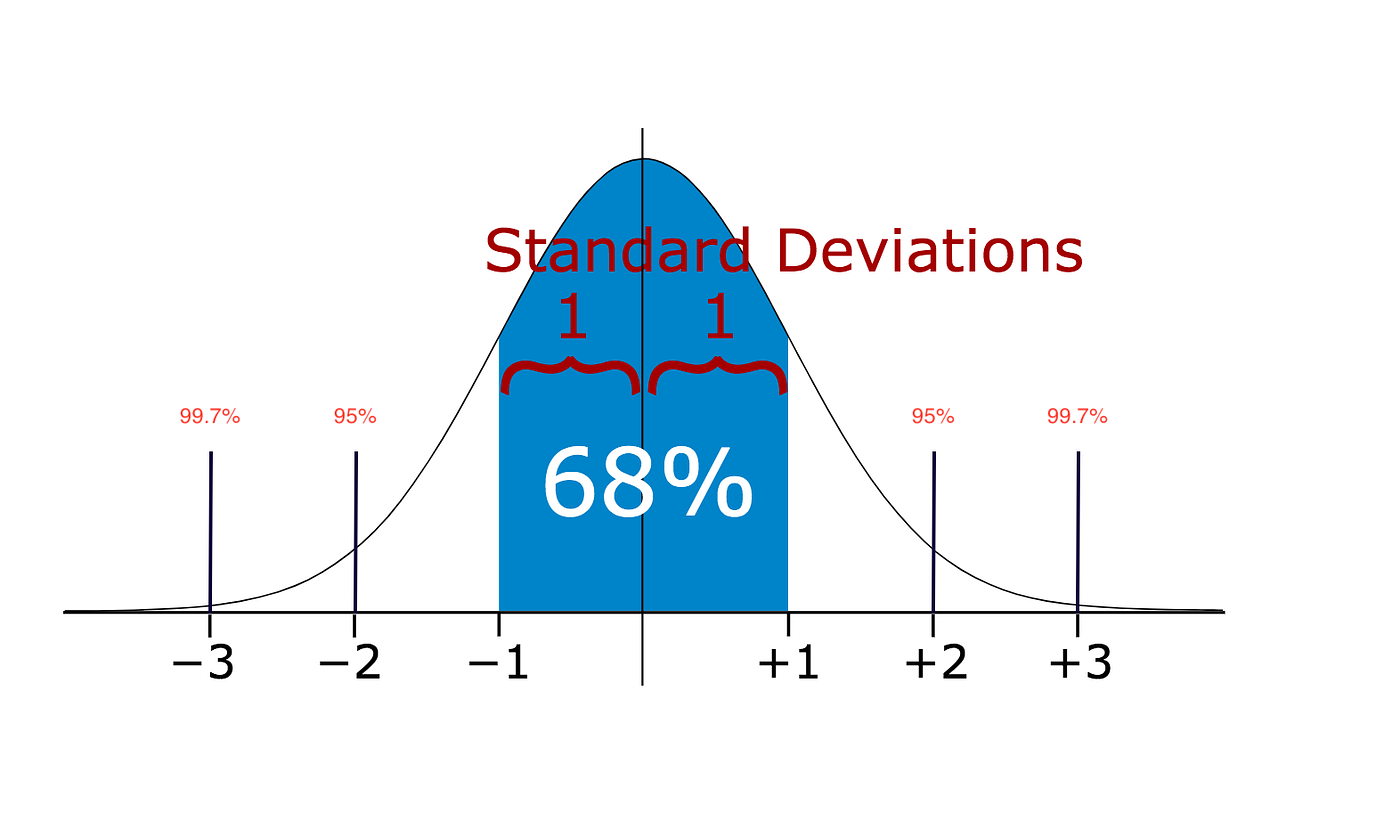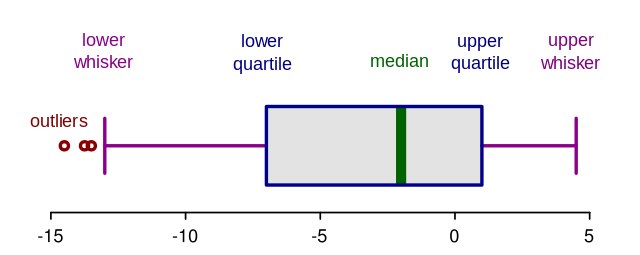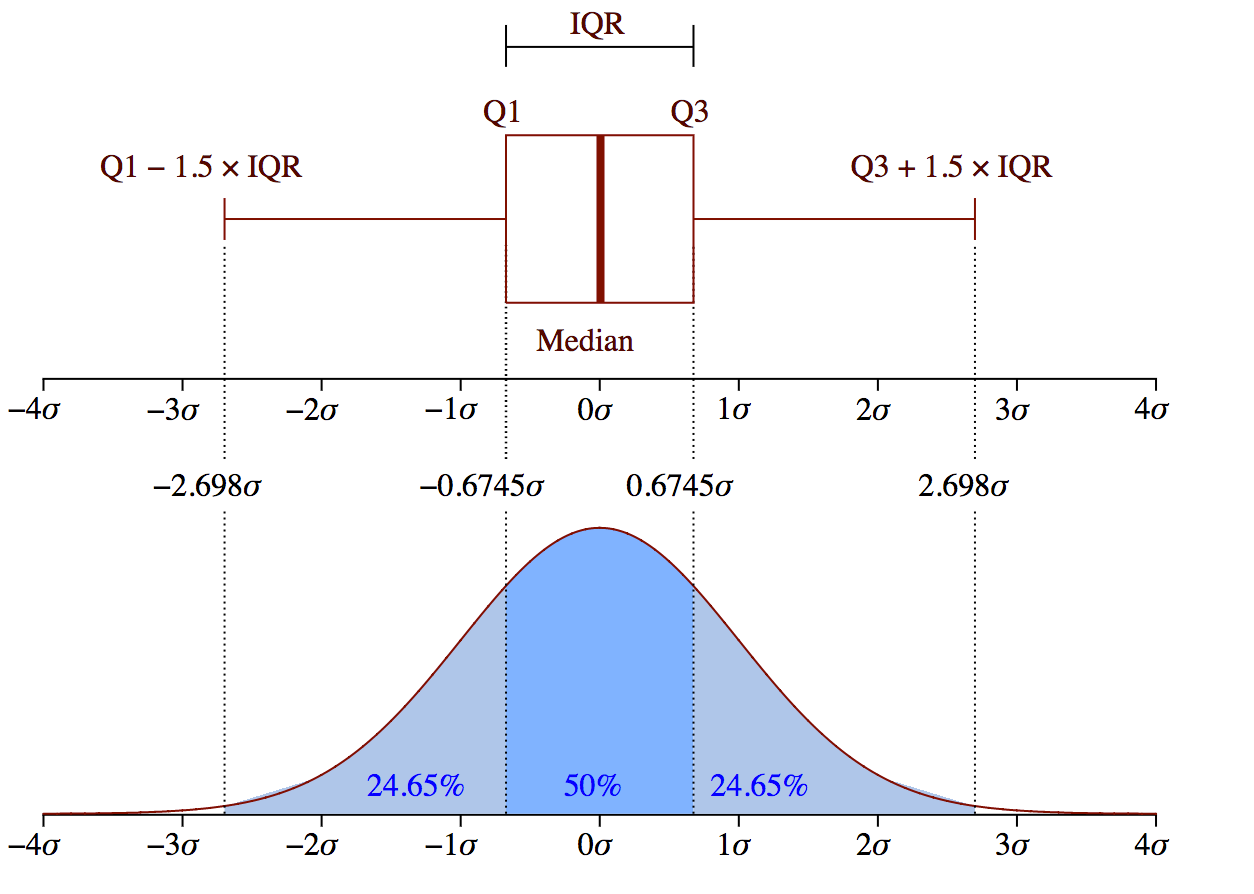# Outliers

Published:

This post covers detecting outliers.

# Outliers

• https://towardsdatascience.com/5-ways-to-detect-outliers-that-every-data-scientist-should-know-python-code-70a54335a623

import numpy as np
import seaborn as sns

import matplotlib.pyplot as plt

from scipy import stats


## Data

# multiply and add by random numbers to get some real values
data = np.random.randn(50000)  * 20 + 20
data


## Method 1 - Scatter Plot

plt.scatter(x=range(len(data)), y=data);


## Method 2 — Standard Deviation

• If a data distribution is approximately normal then about 68% of the data values lie within one standard deviation of the mean and about 95% are within two standard deviations, and about 99.7% lie within three standard deviations

•https://miro.medium.com/max/1400/1*rV7rq7F_uB5gwjzzGJ9VqA.png
def outliers_SD(data):
#define a list to accumlate anomalies
anomalies = []

# Set upper and lower limit to 3 standard deviation
data_std = np.std(data)
data_mean = np.mean(data)

lower_limit  = data_mean - data_std * 3
upper_limit = data_mean + data_std * 3

# Generate outliers
for outlier in data:
if outlier > upper_limit or outlier < lower_limit:
anomalies.append(outlier)
return anomalies

outliers = outliers_SD(data)

plt.scatter(range(len(outliers)), outliers);


## Method 3 — Boxplotshttps://miro.medium.com/max/1280/1*AU07MCIdvUnjskY1XH9auw.pnghttps://miro.medium.com/max/1400/1*J5Xm0X-phCJJ-DKZMZ_88w.png
sns.boxplot(data=data);


## Method 4 - Using Z score

• Z-score is finding the distribution of data where mean is 0 and standard deviation is 1 i.e. normal distribution.

• Re-scale and center the data and look for data points which are too far from zero. These data points which are way too far from zero will be treated as the outliers.

• In most of the cases a threshold of 3 or -3 is used i.e if the Z-score value is greater than or less than 3 or -3 respectively, that data point will be identified as outliers.

• $Z~score = \frac{(Observation — Mean)}{Standard Deviation}$

• $z = \frac{X - \mu}{\sigma}$

def outliers_zscore(data, threshold=3):
outliers=[]

mean = np.mean(data)
std = np.std(data)

for x in data:
z = (x - mean)/std

if np.abs(z) > threshold:
outliers.append(x)

return outliers

outliers = outliers_zscore(data)

plt.scatter(range(len(outliers)), outliers);

def outliers_zscore(data, threshold=3):
z = stats.zscore(data)
z = np.abs(z)
outliers_idx = np.where(z > 3)

outliers = data[outliers_idx]
return outliers

outliers = outliers_zscore(data)

plt.scatter(range(len(outliers)), outliers);


Tags: